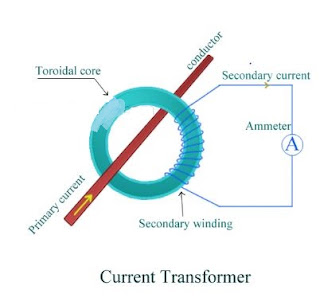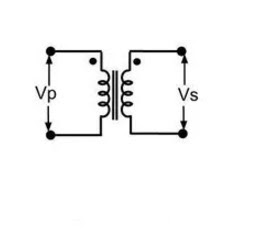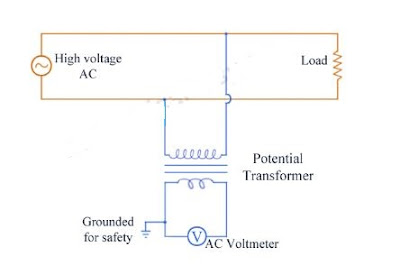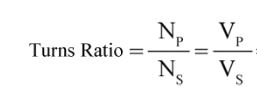# What is an Instrument Transformer : Types and Working

0
413

## Instrument Transformers

### Current Transformers (C.T.) Construction:

#### The secondary of the transformer is made up of a large number of turns of fine wire having small cross-sectional area. This is usually rated for 5 A. This is connected to the coil of normal range ammeter. Through this ammeter we can note down the value of current flowing through the secondary winding of current transformer. Symbolic representation of a current transformer is as shown in the figure.### Working of a current transformer and C.T Ratio:

#### A combination of current transformer and ammeter helps us to find out the higher values of current flowing through line. As discussed we step down the value of line current by increasing the turns in secondary winding. This shows that there is inverse relation between between primary and secondary current and the number of turns of primary and secondary. We connect an ammeter to the secondary winding of current transformer to get secondary current which is shown in the following figure.#### So now we can calculate the value of current through line or primary current from the C.T ratio.### Potential Transformers (P.T) Construction:

#### A potential transformer has two winding’s namely primary and secondary. Primary winding has large number of turns and it is connected in parallel to the line(between line and ground) whose voltage is to be measured. Now the secondary has less number of turns. This is usually rated to 110 V and is connected directly to voltmeter. Through this voltmeter we can note down the value of voltage across the secondary winding of potential transformer.Symbolic representation of a potential transformer is as shown in the figure.### Working of a potential transformer and P.T Ratio:

#### A combination of potential transformer and voltmeter helps us to find out the higher values of potential across the line. As discussed we step down the value of primary voltage by decreasing the turns in secondary winding. This shows that primary and secondary voltage and the number of turns of primary and secondary are directly proportional.We connect an voltmeter across the secondary winding of potential transformer to get secondary voltage which is shown in the following figure.#### So we can calculate the value of line voltage or voltage across the primary of potential transformer from the P.T ratio#### In this post we have learnt about instrument transformers namely current transformer and potential transformer.

READ HERE  What is Current Transformer (CT)? Construction, Working Principle & Types